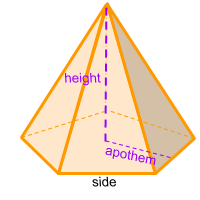Home » Polyhedra » Pyramids » Pentagonal Pyramid

# Volume of a Pentagonal PyramidVolume =
5 + √5/24
× Side ³

## Volume calculator for a pentagonal pyramid

Enter a side of the pentagon that forms the base of the pentagonal pyramid to get the volume automatically.

Other option is to enter the side and apothem of the pentagon that forms the base of the pentagonal pyramid, and the height of the pyramid to get the volume automatically.

Yet another alternative is to enter the side of the pentagon that forms the base of the pentagonal pyramid and the height of the pyramid to get the volume automatically.

If you already know the area of the base you can enter this number along the height of the pyramid to get the volume automatically.

## Description, how many faces, edges and vertices are there in a pentagonal pyramid

The pentagonal pyramid, is a pyramid with a pentagon-shaped base. It has 6 faces, 5 faces are triangles that form the sides and one face considered the base, which in this case it's a pentagon. It has 10 edges and 6 vertices.

## Formula for the volume of a pentagonal pyramid

To calculate the volume of a pentagonal pyramid, we must first know the area of its base. In other words, we must calculate the area of a pentagon (base). To get the area of a pentagon we need to multiply the perimeter of the pentagon by the apothem and then divide the result by 2.
Once we have the area of the base (area of the pentagon), we multiply the result by the height of the pyramid and finally, we divide it by 3.
All of the above is the same as multiplying 5 by the length of one side, then multiply it by the apothem, then by the height and finally divide the result by 6. You can also use the online calculator to calculate the volume of the pentagonal pyramid automatically.

Volume =
5 + √5/24
× Side ³

Formula explanation and alternative formula:

The formula to calculate a pyramid is always the same:

(1) Volume pyramid =
Area base × Height / 3

In this case the area of the base of the pyramid is the area of a pentagon, therefore:

Area base = Area pentagon =
Perimeter × Apothem/2

To calculate the perimeter of a pentagon:

Perimeter = 5 × Side

Now we can replace the Perimeter in the formula (1):

Volume =
5 × Side × Apothem × Height /6

To calculate the apothem:

Apothem =
side/2•tan(α÷2)
, with
α =
360°/5

and to calculate the height:

Height = √(
5-√5/10
) × Side

Finally, if we replace all the variables. We found another way to calculate the volume of a pentagonal pyramid without knowing the value of the apothem

Volume =
5 × side ² × height / 12 × Tan(36°)
=
5 + √5/24
× Side ³

Share